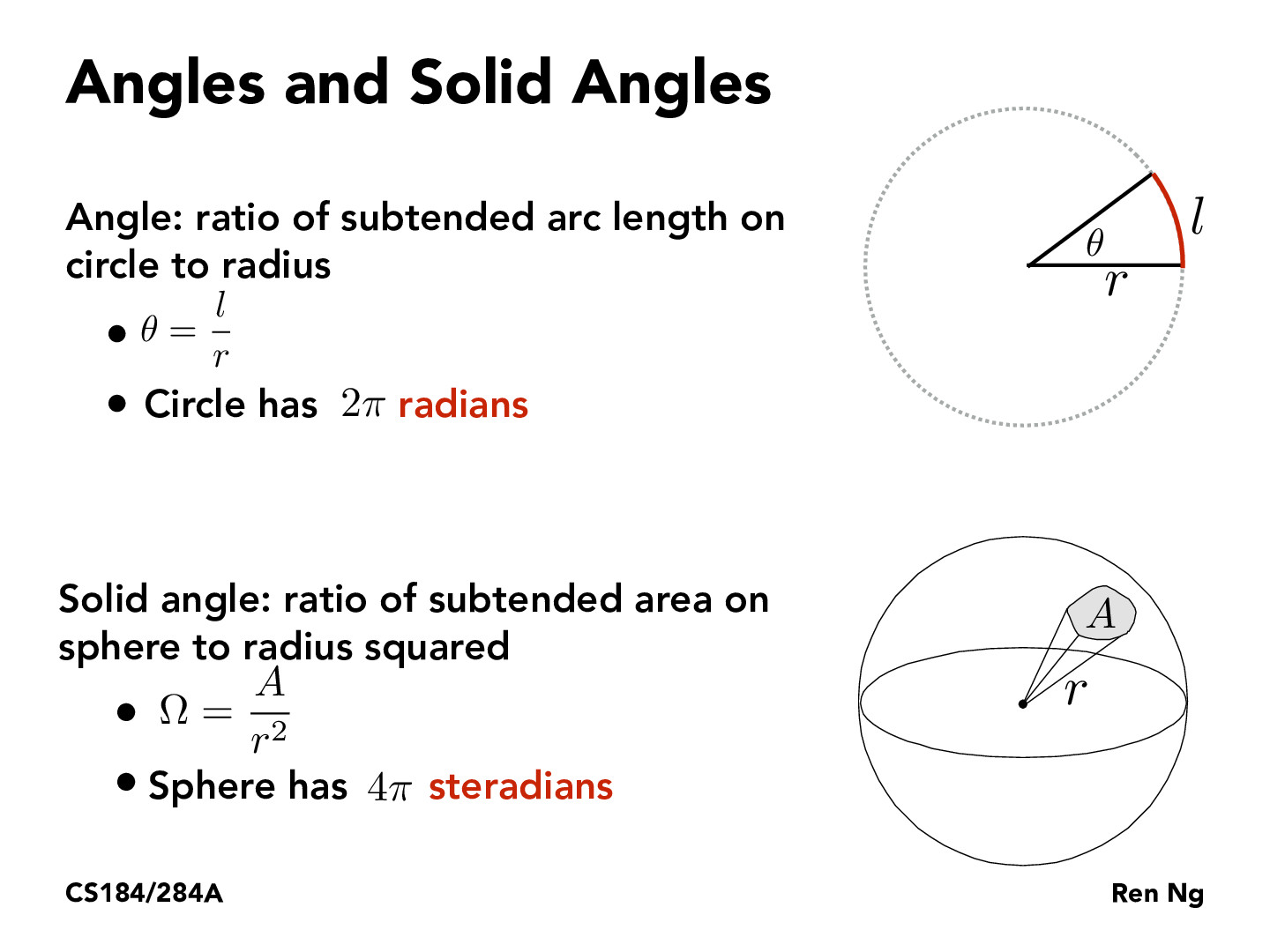Lecture 11: Radiometry and Photometry (12)sungpark98

Is there any visual way of thinking of how much a steradian is? Is it similar to radians? For example with radians, we know that pi radians should be perpendicular and 2 pi is straight lines. Is it similar with steradians?FLinesse

Not sure if this helps, but since radians and steradians are dimensionless numbers I just think of those numbers as proportions out of the whole. For example, pi steradians would be pi/4pi, equivalent to 1/4th of a sphere and 2pi steradians would be 2pi/4pi, equivalent to 1/2th of a spherejinwoopark1673

@sungpart98, since we are given that a sphere has 4pi steradians (4pi r^2/r^2=4pi), we can think of steradian as the area of the portion of a sphere with radius reduced to 1 proportionally.rlna

This Quora post helped me grasp the concept of steradians in relation to radians a bit more clearly. There are a few explanations with graphics on what a solid angle is and how it is used. https://www.quora.com/What-is-solid-anglejamesyc23

There's also a notion of square degrees (although it seems to be much less commonly used), with the conversion (pi/180)^2 steradians = 1 square degree -- similar to how pi/180 radians = 1 degree.

You must be enrolled in the course to comment